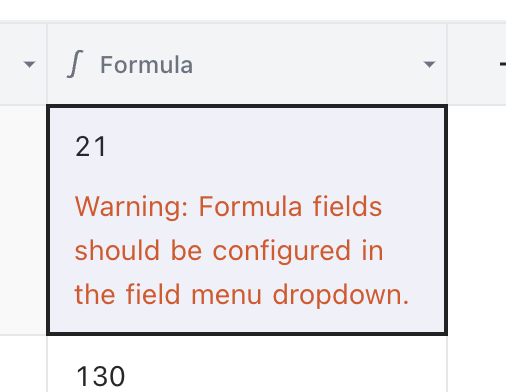Version: Latest

# Formulas• Click on '+' (Add column)
• Populate column Name
• Select column Type as 'Formula'
• Insert required formula
• You can use explicit numerical values/ strings as needed, e.g. `123` (numeric) or `"123"` (string).
• You can reference column names in equation with `{}`, e.g. `{column_name}`, if the column name conflicts with literals
• Table below lists supported formula & associated syntax
• Nested formula (formula equation referring to another formula column) is supported
• Click on 'Save'

## Editing formula column​

Unlike other column types, formula cells cannot be modified by double-clicking since the value is generated based on the formula. Instead, the vaule can be changed by updating the formula in the column setting.## Available Formula Features​

### Numeric Functions​

NameSyntaxSampleOutput
ABS`ABS(value)``ABS({Column})`Absolute value of the input parameter
ADD`ADD(value1,[value2,...])``ADD({Column1}, {Column2})`Sum of input parameters
AVG`AVG(value1,[value2,...])``AVG({Column1}, {Column2})`Average of input parameters
CEILING`CEILING(value)``CEILING({Column})`Rounded next largest integer value of input parameter
EXP`EXP(value)``EXP({Column})`Exponential value of input parameter (`e^x`)
FLOOR`FLOOR(value)``FLOOR({Column})`Rounded largest integer less than or equal to input parameter
INT`INT(value)``INT({Column})`Integer value of input parameter
LOG`LOG([base], value)``LOG(10, {Column})`Logarithm of input parameter to the base (default = e) specified
MAX`MAX(value1,[value2,...])``MAX({Column1}, {Column2}, {Column3})`Maximum value amongst input parameters
MIN`MIN(value1,[value2,...])``MIN({Column1}, {Column2}, {Column3})`Minimum value amongst input parameters
MOD`MOD(value1, value2)``MOD({Column}, 2)`Remainder after integer division of input parameters
POWER`POWER(base, exponent)``POWER({Column}, 3)``base` to the `exponent` power, as in `base ^ exponent`
ROUND`ROUND(value, precision)``ROUND({Column}, 3)`Round input `value` to decimal place specified by `precision` (Nearest integer if `precision` not provided)
SQRT`SQRT(value)``SQRT({Column})`Square root of the input parameter

### Numeric Operators​

OperatorSampleDescription
`+``{Column1} + {Column2} + 2`Addition of numeric values
`-``{Column1} - {Column2}`Subtraction of numeric values
`*``{Column1} * {Column2}`Multiplication of numeric values
`/``{Column1} / {Column2}`Division of numeric values

Tip : To change the order of arithmetic operation, you can use round bracket parenthesis ().
Example: ({Column1} + ({Column2} * {Column3}) / (3 - \$Column4\$ ))

### String Functions​

NameSyntaxSampleOutput
CONCAT`CONCAT(str1, [str2,...])``CONCAT({Column1}, ' ', {Column2})`Concatenated string of input parameters
LEFT`LEFT(str1, n)``LEFT({Column}, 3)``n` characters from the beginning of input parameter
LEN`LEN(str)``LEN({Column})`Input parameter character length
LOWER`LOWER(str)``LOWER({Column})`Lower case converted string of input parameter
MID`MID(str, position, [count])``MID({Column}, 3, 2)`Alias for `SUBSTR`
REPEAT`REPEAT(str, count)``REPEAT({Column}, 2)`Specified copies of the input parameter string concatenated together
REPLACE`REPLACE(str, srchStr, rplcStr)``REPLACE({Column}, 'int', 'num')`String, after replacing all occurrences of `srchStr` with `rplcStr`
RIGHT`RIGHT(str, n)``RIGHT({Column}, 3)``n` characters from the end of input parameter
SEARCH`SEARCH(str, srchStr)``SEARCH({Column}, 'str')`Index of `srchStr` specified if found, 0 otherwise
SUBSTR`SUBTR(str, position, [count])``SUBSTR({Column}, 3, 2)`Substring of length 'count' of input string, from the postition specified
TRIM`TRIM(str)``TRIM({Column})`Remove trailing and leading whitespaces from input parameter
UPPER`UPPER(str)``UPPER({Column})`Upper case converted string of input parameter
URL`URL(str)``URL({Column})`Convert to a hyperlink if it is a valid URL

### Date Functions​

NameSyntaxSampleOutputRemark
NOW`NOW()``NOW()`2022-05-19 17:20:43Returns the current time and day
`IF(NOW() < {DATE_COL}, "true", "false")``IF(NOW() < date, "true", "false")`If current date is less than {DATE_COL}, it returns true. Otherwise, it returns false.DateTime columns and negative values are supported.
DATEADD`DATEADD(date \| datetime, value, ["day" \| "week" \| "month" \| "year"])``DATEADD(date, 1, 'day')`Supposing {DATE_COL} is 2022-03-14. The result is 2022-03-15.DateTime columns and negative values are supported. Example: `DATEADD(DATE_TIME_COL, -1, 'day')`
`DATEADD(date, 1, 'week')`Supposing {DATE_COL} is 2022-03-14 03:14. The result is 2022-03-21 03:14.DateTime columns and negative values are supported. Example: `DATEADD(DATE_TIME_COL, -1, 'week')`
`DATEADD(date, 1, 'month')`Supposing {DATE_COL} is 2022-03-14 03:14. The result is 2022-04-14 03:14.DateTime columns and negative values are supported. Example: `DATEADD(DATE_TIME_COL, -1, 'month')`
`DATEADD(date, 1, 'year')`Supposing {DATE_COL} is 2022-03-14 03:14. The result is 2023-03-14 03:14.DateTime columns and negative values are supported. Example: `DATEADD(DATE_TIME_COL, -1, 'year')`
`IF(NOW() < DATEADD(date,10,'day'), "true", "false")`If the current date is less than {DATE_COL} plus 10 days, it returns true. Otherwise, it returns false.DateTime columns and negative values are supported.
`IF(NOW() < DATEADD(date,10,'day'), "true", "false")`If the current date is less than {DATE_COL} plus 10 days, it returns true. Otherwise, it returns false.DateTime columns and negative values are supported.
DATETIME_DIFF`DATETIME_DIFF(date, date, ["milliseconds" \| "ms" \| "seconds" \| "s" \| "minutes" \| "m" \| "hours" \| "h" \| "days" \| "d" \| "weeks" \| "w" \| "months" \| "M" \| "quarters" \| "Q" \| "years" \| "y"])``DATETIME_DIFF("2022/10/14", "2022/10/15", "second")`Supposing {DATE_COL_1} is 2017-08-25 and {DATE_COL_2} is 2011-08-25. The result is 86400.Compares two dates and returns the difference in the unit specified. Positive integers indicate the second date being in the past compared to the first and vice versa for negative ones.
`WEEKDAY(NOW(), "sunday")`If today is Monday, it returns 1Get the week day of NOW() with the first day set as sunday
WEEKDAY`WEEKDAY(date, [startDayOfWeek])``WEEKDAY(NOW())`If today is Monday, it returns 0Returns the day of the week as an integer between 0 and 6 inclusive starting from Monday by default. You can optionally change the start day of the week by specifying in the second argument
`WEEKDAY(NOW(), "sunday")`If today is Monday, it returns 1Get the week day of NOW() with the first day set as sunday

### Logical Operators​

OperatorSampleDescription
`<``{Column1} < {Column2}`Less than
`>``{Column1} > {Column2}`Greater than
`<=``{Column1} <= {Column2}`Less than or equal to
`>=``{Column1} >= {Column2}`Greater than or equal to
`==``{Column1} == {Column2}`Equal to
`!=``{Column1} != {Column2}`Not equal to

### Conditional Expressions​

NameSyntaxSampleOutput
IF`IF(expr, successCase, elseCase)``IF({Column} > 1, Value1, Value2)`successCase if `expr` evaluates to TRUE, elseCase otherwise
SWITCH`SWITCH(expr, [pattern, value, ..., default])``SWITCH({Column}, 1, 'One', 2, 'Two', '--')`Switch case value based on `expr` output
AND`AND(expr1, [expr2,...])``AND({Column} > 2, {Column} < 10)`TRUE if all `expr` evaluate to TRUE
OR`OR(expr1, [expr2,...])``OR({Column} > 2, {Column} < 10)`TRUE if at least one `expr` evaluates to TRUE

Logical operators, along with Numerical operators can be used to build conditional `expressions`.

Examples:

``IF({marksSecured} > 80, "GradeA", "GradeB")  ``
``SWITCH({quarterNumber},      1, 'Jan-Mar',    2, 'Apr-Jun',    3, 'Jul-Sep',    4, 'Oct-Dec',    'INVALID')``